# Image Approximation with Fourier and Wavelets¶

Important: Please read the installation page for details about how to install the toolboxes. $\newcommand{\dotp}{\langle #1, #2 \rangle}$ $\newcommand{\enscond}{\lbrace #1, #2 \rbrace}$ $\newcommand{\pd}{ \frac{ \partial #1}{\partial #2} }$ $\newcommand{\umin}{\underset{#1}{\min}\;}$ $\newcommand{\umax}{\underset{#1}{\max}\;}$ $\newcommand{\umin}{\underset{#1}{\min}\;}$ $\newcommand{\uargmin}{\underset{#1}{argmin}\;}$ $\newcommand{\norm}{\|#1\|}$ $\newcommand{\abs}{\left|#1\right|}$ $\newcommand{\choice}{ \left\{ \begin{array}{l} #1 \end{array} \right. }$ $\newcommand{\pa}{\left(#1\right)}$ $\newcommand{\diag}{{diag}\left( #1 \right)}$ $\newcommand{\qandq}{\quad\text{and}\quad}$ $\newcommand{\qwhereq}{\quad\text{where}\quad}$ $\newcommand{\qifq}{ \quad \text{if} \quad }$ $\newcommand{\qarrq}{ \quad \Longrightarrow \quad }$ $\newcommand{\ZZ}{\mathbb{Z}}$ $\newcommand{\CC}{\mathbb{C}}$ $\newcommand{\RR}{\mathbb{R}}$ $\newcommand{\EE}{\mathbb{E}}$ $\newcommand{\Zz}{\mathcal{Z}}$ $\newcommand{\Ww}{\mathcal{W}}$ $\newcommand{\Vv}{\mathcal{V}}$ $\newcommand{\Nn}{\mathcal{N}}$ $\newcommand{\NN}{\mathcal{N}}$ $\newcommand{\Hh}{\mathcal{H}}$ $\newcommand{\Bb}{\mathcal{B}}$ $\newcommand{\Ee}{\mathcal{E}}$ $\newcommand{\Cc}{\mathcal{C}}$ $\newcommand{\Gg}{\mathcal{G}}$ $\newcommand{\Ss}{\mathcal{S}}$ $\newcommand{\Pp}{\mathcal{P}}$ $\newcommand{\Ff}{\mathcal{F}}$ $\newcommand{\Xx}{\mathcal{X}}$ $\newcommand{\Mm}{\mathcal{M}}$ $\newcommand{\Ii}{\mathcal{I}}$ $\newcommand{\Dd}{\mathcal{D}}$ $\newcommand{\Ll}{\mathcal{L}}$ $\newcommand{\Tt}{\mathcal{T}}$ $\newcommand{\si}{\sigma}$ $\newcommand{\al}{\alpha}$ $\newcommand{\la}{\lambda}$ $\newcommand{\ga}{\gamma}$ $\newcommand{\Ga}{\Gamma}$ $\newcommand{\La}{\Lambda}$ $\newcommand{\si}{\sigma}$ $\newcommand{\Si}{\Sigma}$ $\newcommand{\be}{\beta}$ $\newcommand{\de}{\delta}$ $\newcommand{\De}{\Delta}$ $\newcommand{\phi}{\varphi}$ $\newcommand{\th}{\theta}$ $\newcommand{\om}{\omega}$ $\newcommand{\Om}{\Omega}$

This numerical tour overviews the use of Fourier and wavelets for image approximation.

In :
using PyPlot
using NtToolBox
arequire("NtToolBox")

WARNING: Base.UTF8String is deprecated, use String instead.
WARNING: Base.UTF8String is deprecated, use String instead.
WARNING: Base.UTF8String is deprecated, use String instead.
WARNING: Base.UTF8String is deprecated, use String instead.
WARNING: Base.UTF8String is deprecated, use String instead.
WARNING: Base.UTF8String is deprecated, use String instead.
likely near In:4
WARNING: Base.UTF8String is deprecated, use String instead.
likely near In:4
WARNING: Base.UTF8String is deprecated, use String instead.
likely near In:4
WARNING: Base.UTF8String is deprecated, use String instead.
likely near In:4
WARNING: Base.UTF8String is deprecated, use String instead.
likely near In:4
WARNING: Base.UTF8String is deprecated, use String instead.
likely near In:4
WARNING: Base.UTF8String is deprecated, use String instead.
likely near In:4
WARNING: Base.UTF8String is deprecated, use String instead.
likely near In:4
WARNING: Base.UTF8String is deprecated, use String instead.
likely near In:4
WARNING: Base.UTF8String is deprecated, use String instead.
likely near In:4
WARNING: Base.UTF8String is deprecated, use String instead.
likely near In:4
WARNING: Base.UTF8String is deprecated, use String instead.
likely near In:4
WARNING: Base.UTF8String is deprecated, use String instead.
likely near In:4
WARNING: Base.UTF8String is deprecated, use String instead.
likely near In:4
WARNING: Base.UTF8String is deprecated, use String instead.
likely near In:4
WARNING: Base.UTF8String is deprecated, use String instead.
likely near In:4
WARNING: Base.UTF8String is deprecated, use String instead.
likely near In:4
WARNING: Base.UTF8String is deprecated, use String instead.
likely near In:4
WARNING: Base.UTF8String is deprecated, use String instead.
likely near In:4
WARNING: Base.UTF8String is deprecated, use String instead.
likely near In:4
WARNING: Base.UTF8String is deprecated, use String instead.
likely near In:4
WARNING: Base.UTF8String is deprecated, use String instead.
likely near In:4
WARNING: Base.UTF8String is deprecated, use String instead.
likely near In:4
WARNING: Base.UTF8String is deprecated, use String instead.
likely near In:4
WARNING: Base.UTF8String is deprecated, use String instead.
likely near In:4
WARNING: Base.UTF8String is deprecated, use String instead.
likely near In:4
WARNING: Base.UTF8String is deprecated, use String instead.
likely near In:4
WARNING: Base.UTF8String is deprecated, use String instead.
likely near In:4
WARNING: Base.UTF8String is deprecated, use String instead.
likely near In:4
WARNING: Base.UTF8String is deprecated, use String instead.
likely near In:4
WARNING: Base.UTF8String is deprecated, use String instead.
likely near In:4
WARNING: Base.UTF8String is deprecated, use String instead.
likely near In:4
WARNING: Base.UTF8String is deprecated, use String instead.
likely near In:4
WARNING: Base.UTF8String is deprecated, use String instead.
likely near In:4
WARNING: Base.UTF8String is deprecated, use String instead.
likely near In:4
WARNING: Base.UTF8String is deprecated, use String instead.
likely near In:4
WARNING: Base.UTF8String is deprecated, use String instead.
likely near In:4
WARNING: Base.UTF8String is deprecated, use String instead.
likely near In:4
WARNING: Base.UTF8String is deprecated, use String instead.
likely near In:4
WARNING: Base.UTF8String is deprecated, use String instead.
likely near In:4
in depwarn(::String, ::Symbol) at .\deprecated.jl:64
in #parse_file#6(::Array{Any,1}, ::Function, ::String) at C:\Users\Ayman\.julia\v0.5\Autoreload\src\files.jl:63
in execute_request(::ZMQ.Socket, ::IJulia.Msg) at C:\Users\Ayman\.julia\v0.5\IJulia\src\execute_request.jl:157
in eventloop(::ZMQ.Socket) at C:\Users\Ayman\.julia\v0.5\IJulia\src\eventloop.jl:8
WARNING: replacing module NtToolBox


Note: to measure the error of an image $f$ with its approximation $f_M$, we use the SNR measure, defined as

$$\text{SNR}(f,f_M) = -20\log_{10} \pa{ \frac{ \norm{f-f_M} }{ \norm{f} } },$$

which is a quantity expressed in decibels (dB). The higer the SNR, the better the quality.

First we load an image $f \in \RR^N$ of $N = N_0 \times N_0$ pixels.

In :
n0 = 512

WARNING: Base.UTF8String is deprecated, use String instead.
likely near C:\Users\Ayman\.julia\v0.5\IJulia\src\kernel.jl:31
WARNING: Base.UTF8String is deprecated, use String instead.
likely near C:\Users\Ayman\.julia\v0.5\IJulia\src\kernel.jl:31
WARNING: Base.UTF8String is deprecated, use String instead.
likely near C:\Users\Ayman\.julia\v0.5\IJulia\src\kernel.jl:31
WARNING: Base.UTF8String is deprecated, use String instead.
likely near C:\Users\Ayman\.julia\v0.5\IJulia\src\kernel.jl:31
WARNING: Base.UTF8String is deprecated, use String instead.
likely near C:\Users\Ayman\.julia\v0.5\IJulia\src\kernel.jl:31
WARNING: Base.UTF8String is deprecated, use String instead.
likely near C:\Users\Ayman\.julia\v0.5\IJulia\src\kernel.jl:31


Display the original image.

In :
figure(figsize = (5,5))
imageplot(f, "Image f")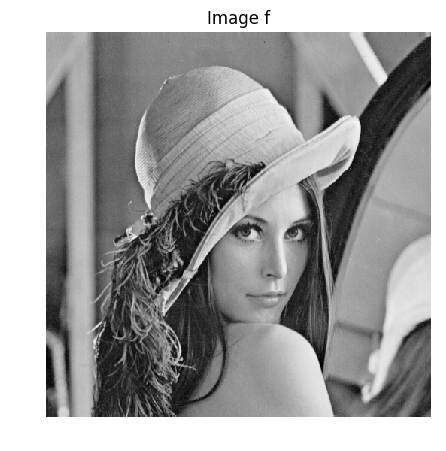Out:
PyObject <matplotlib.text.Text object at 0x000000001E9D20B8>

Display a zoom in the middle.

In :
figure(figsize = (5,5))
imageplot(f[Int(n0/2 - 32) : Int(n0/2 + 32), Int(n0/2 - 32) : Int(n0/2 + 32)], "Zoom")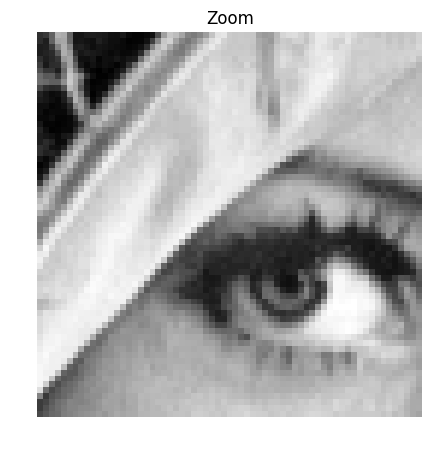Out:
PyObject <matplotlib.text.Text object at 0x000000001E7DA240>

An image is a 2D array, it can be modified as a matrix.

In :
figure(figsize = (8,8))
imageplot(-f, "-f", [1, 2, 1])
imageplot(f[end:-1:1, :], "Flipped", [1, 2, 2])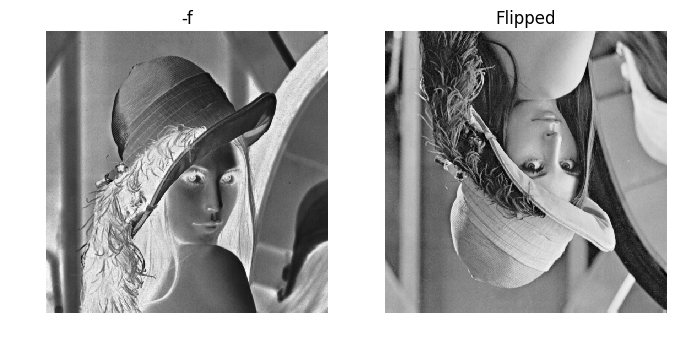Out:
PyObject <matplotlib.text.Text object at 0x000000001EF47898>

Blurring is achieved by computing a convolution $f \star h$ with a kernel $h$.

Compute the low pass kernel.

In :
k = 9; #size of the kernel
h = ones(k, k)
h = h/sum(h); #normalize

Out:
9×9 Array{Float64,2}:
0.0123457  0.0123457  0.0123457  …  0.0123457  0.0123457  0.0123457
0.0123457  0.0123457  0.0123457     0.0123457  0.0123457  0.0123457
0.0123457  0.0123457  0.0123457     0.0123457  0.0123457  0.0123457
0.0123457  0.0123457  0.0123457     0.0123457  0.0123457  0.0123457
0.0123457  0.0123457  0.0123457     0.0123457  0.0123457  0.0123457
0.0123457  0.0123457  0.0123457  …  0.0123457  0.0123457  0.0123457
0.0123457  0.0123457  0.0123457     0.0123457  0.0123457  0.0123457
0.0123457  0.0123457  0.0123457     0.0123457  0.0123457  0.0123457
0.0123457  0.0123457  0.0123457     0.0123457  0.0123457  0.0123457

Compute the convolution $f \star h$.

In :
fh = conv2(Array{Float64, 2}(f), h);


Display.

In :
figure(figsize = (5,5))
imageplot(fh, "Blurred image")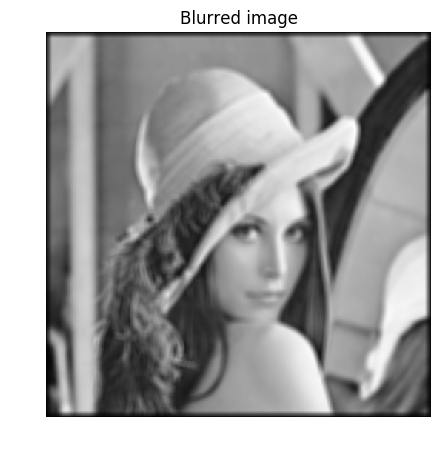Out:
PyObject <matplotlib.text.Text object at 0x000000001F218C50>

## Fourier Transform¶

The Fourier orthonormal basis is defined as $$\psi_m(k) = \frac{1}{\sqrt{N}}e^{\frac{2i\pi}{N_0} \dotp{m}{k} }$$ where $0 \leq k_1,k_2 < N_0$ are position indexes, and $0 \leq m_1,m_2 < N_0$ are frequency indexes.

The Fourier transform $\hat f$ is the projection of the image on this Fourier basis

$$\hat f(m) = \dotp{f}{\psi_m}.$$

The Fourier transform is computed in $O(N \log(N))$ operation using the FFT algorithm (Fast Fourier Transform). Note the normalization by $\sqrt{N}=N_0$ to make the transform orthonormal.

In :
F = plan_fft(f)
F = (F*f)/n0;


We check this conservation of the energy.

In :
println(@sprintf("Energy of Image:   %f", norm(f)))
println(@sprintf("Energy of Fourier:   %f", norm(F)))

Energy of Image:   262.554108
Energy of Fourier:   262.554138


Compute the logarithm of the Fourier magnitude $\log\left(\abs{\hat f(m)} + \epsilon\right)$, for some small $\epsilon$.

In :
L = fftshift(log(abs(F) + 1e-1));


Display. Note that we use the function fftshift to put the 0 low frequency in the middle.

In :
figure(figsize = (5,5))
imageplot(L, "Log(Fourier transform)")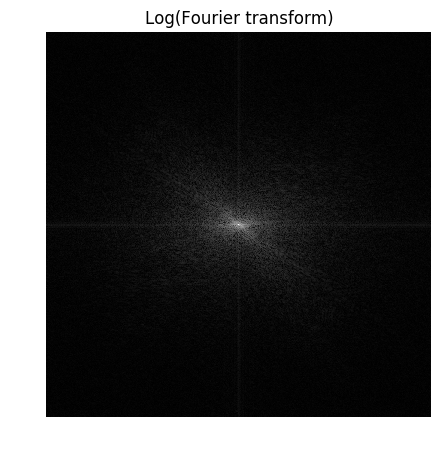Out:
PyObject <matplotlib.text.Text object at 0x00000000244FA978>

## Linear Fourier Approximation¶

An approximation is obtained by retaining a certain set of index $I_M$

$$f_M = \sum_{ m \in I_M } \dotp{f}{\psi_m} \psi_m.$$

Linear approximation is obtained by retaining a fixed set $I_M$ of $M = \abs{I_M}$ coefficients. The important point is that $I_M$ does not depend on the image $f$ to be approximated.

For the Fourier transform, a low pass linear approximation is obtained by keeping only the frequencies within a square.

$$I_M = \enscond{m=(m_1,m_2)}{ -q/2 \leq m_1,m_2 < q/2 }$$

where $q = \sqrt{M}$.

This can be achieved by computing the Fourier transform, setting to zero the $N-M$ coefficients outside the square $I_M$ and then inverting the Fourier transform.

Number $M$ of kept coefficients.

In :
M = Base.div(n0^2, 64);


Exercise 1

Perform the linear Fourier approximation with $M$ coefficients. Store the result in the variable $f_M$.

In :
include("NtSolutions/introduction_4_fourier_wavelets/exo1.jl")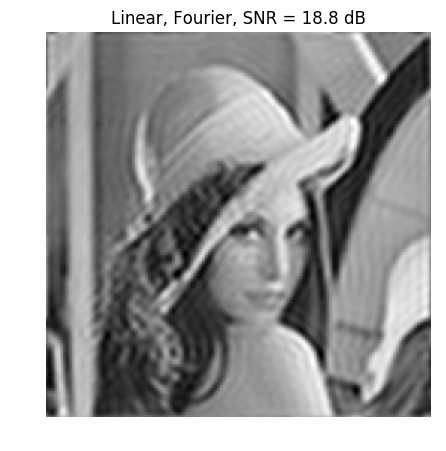Out:
PyObject <matplotlib.text.Text object at 0x0000000024A79908>
In :
## Insert your code here.


Compare two 1D profile (lines of the image). This shows the strong ringing artifact of the linea approximation.

In :
figure(figsize = (7, 6))

subplot(2, 1, 1)
plot(f[: , Base.div(n0, 2)])
xlim(0, n0)
title("f")

subplot(2, 1, 2)
plot(fM[: , Base.div(n0, 2)])
xlim(0, n0)
title("f_M")

show()